# Civil Engineering - UPSC Civil Service Exam Questions

46.
At the same mean velocity, what is the ratio of head loss per unit length for a sewer pipe flowing full to that for the same pipe flowing half full ?
2.0
1.7
1.0
0.6
Explanation:
No answer description is available. Let's discuss.

47.
A heavy elastic ball drops from the ceiling of a room, and after rebounding once from the floor, reaches a height equal to three-fourth of that of the ceiling. The coefficient of restitution will be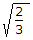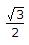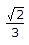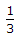Explanation:
No answer description is available. Let's discuss.

48.
A beam of rectangular section having simply supported span L, is subject to a concentrated load at its mid-span. What is the length of elasto-plastic zone of the plastic hinge ?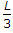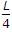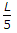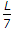Explanation:
No answer description is available. Let's discuss.

49.
Consider the following statement: High early strength of cement is obtained as a result of
1. fine grinding.
2. decreasing the lime content
3. burning at higher temperatures
4. increasing the quantity of gypsum.
Of these statements :
1 and 2 are correct
1 and 3 are correct
2, 3 and 4 are correct
1, 3 and 4 are correct
Explanation:
No answer description is available. Let's discuss.

50.
A prismatic beam is shown in the figure. Consider the following statements :
1. The structure is unstable.
2. The bending moment is zero at supports and internal hinge.
3. It is mechanism.
4. It is statically indeterminate.
Which of the statements given above is/are correct ?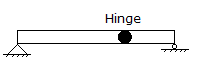1, 2, 3 and 4
1, 2 and 3
1 and 2
3 and 4